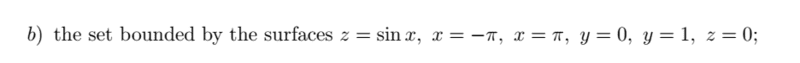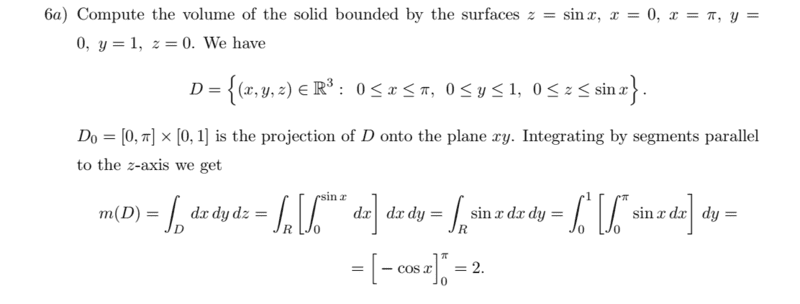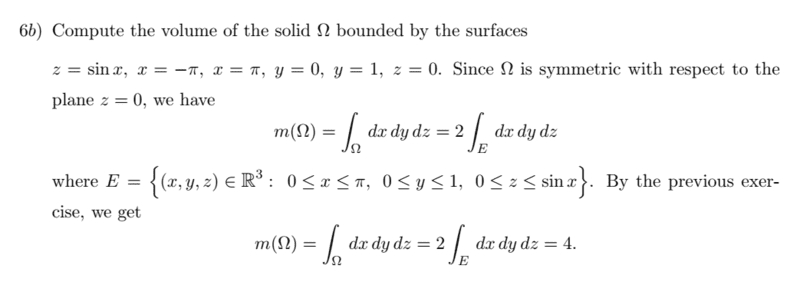# Why this triple integral is not null?

• Amaelle
In summary, the conversation discusses the concept of definite integration and the computation of volume using this technique. The disagreement arises when integrating sin(x) from 0 to pi, as one person argues that the integral should be 0 over a symmetric region while the other points out that the integration domain is not symmetric relative to zero. The difference between signed and unsigned areas is also mentioned. Ultimately, it is concluded that the area of a shape, such as a circle, is not always zero even if half of it is above the x-axis and half is below. f

#### Amaelle

Homework Statement
look at the image
Relevant Equations
integrating impair function over symmetric region
Greetings
here is my integral
Compute the volume of the solidand here is the solution (that I don't agree with)So as you can see they started integrating sinx from 0 to pi and then multiplied everything by two! for me sin(x) is an odd function and it's integral should be 0 over symmetric region!

I am confused. Definite integration is a technique to find the area under the curve. Sin(x) is completely above the x-axis between (0, Pi). So the value isn't 0.

•Amaelle
By definition, volume is positive and all its pieces have positive volume. So the fact that sin() is odd forces you to consider the negative part as an additional positive volume.

••Amaelle and Steve4Physics
Homework Statement:: look at the image
Relevant Equations:: integrating impair function over symmetric region

So as you can see they started integrating sinx from 0 to pi and then multiplied everything by two! for me sin(x) is an odd function and it's integral should be 0 over symmetric region!
sin(x) is odd relative to zero. Your integration domain is not symmetric relative to zero. Hence, your argument does not apply.

•Amaelle
So as you can see they started integrating sinx from 0 to pi and then multiplied everything by two! for me sin(x) is an odd function and it's integral should be 0 over symmetric region!
The area of (say) a circle, centred somewhere on the x-axis, is not zero - even though half the area is above the x-axis and half is below.

Beware of the difference between signed and unsigned areas!

••Amaelle and FactChecker
The area of (say) a circle, centred somewhere on the x-axis, is not zero - even though half the area is above the x-axis and half is below.

Beware of the difference between signed and unsigned areas!
thank you I got it!

The area of (say) a circle, centred somewhere on the x-axis, is not zero - even though half the area is above the x-axis and half is below.

Beware of the difference between signed and unsigned areas!
sin(x) is odd relative to zero. Your integration domain is not symmetric relative to zero. Hence, your argument does not apply.
thank you very much! I understood the difference now!

•berkeman